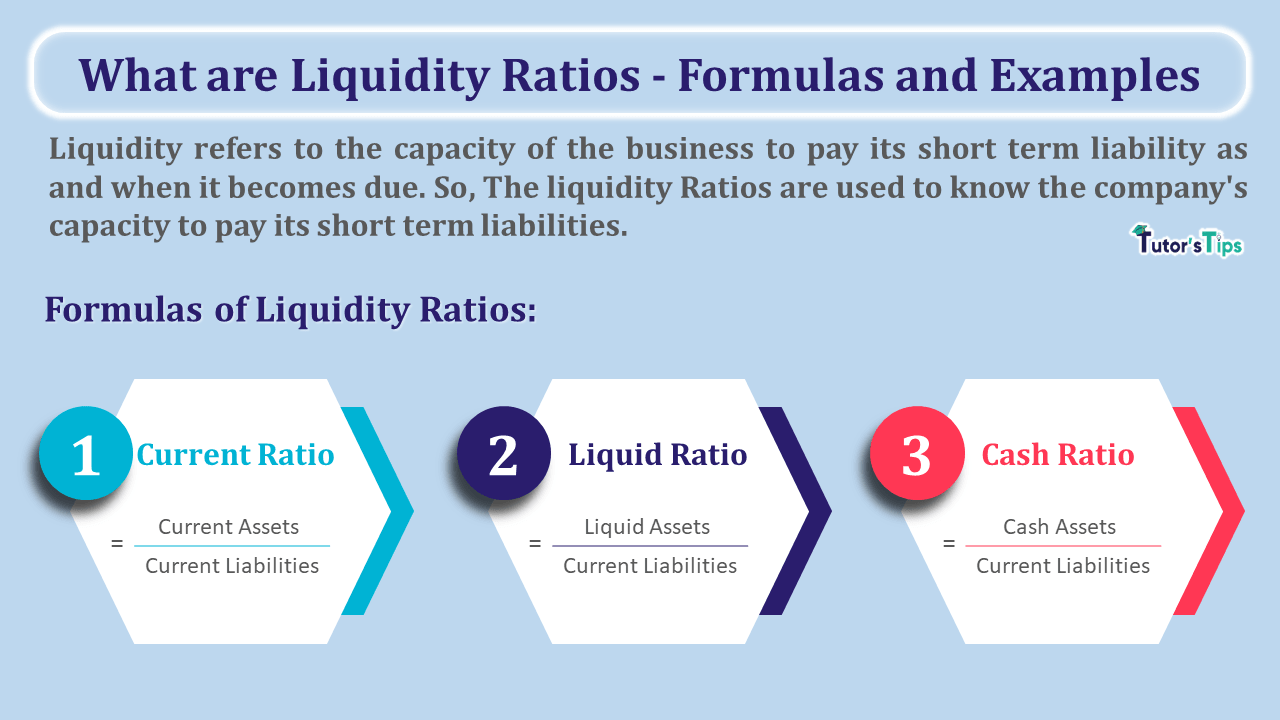# What are Liquidity Ratios – Formulas and ExamplesWhat are Liquidity Ratios - Formulas and Examples

Liquidity Ratios show the capacity of the company to repay its current liabilities by realising the current assets. It is the basic ratio of the accounting ratios.

## Meaning of Liquidity Ratios:

Liquidity refers to the capacity of the business to pay its short term liability as and when it becomes due. So, The liquidity Ratios are used to know the company’s capacity to pay its short term liabilities.

To pay the short term liabilities means paid the total due amount of short term liabilities by realising amount from the company’s short term assets.

## Why do we need to Calculate Liquidity Ratios?

Liquidity ratio provides us with information about the liquidity of the business. The high liquidity ratio ensures that the company is in a good position to meet its financial obligation and the low liquidity ratio shows the low capacity of the firm to meet its financial obligation and it will lead to bankruptcy and fall down the credit rating of the business.

## The formula of Liquidity Ratios:

To Check out the liquidity of the business we can use the following three formulas:

1. Current Ratio
2. Quick or liquid Ratio
3. Absolute liquid or cash Ratio:

### 1. Current Ratio:

The current ratio is used to compare the current assets with current liabilities of the business.

###### Formula to calculate Current Ratio:
 Current Ratio = Current Assets Current Liabilities

### 2. Quick or liquid Ratio

The Quick or liquid Ratio is used to compare the Liquid assets with current liabilities of the business.

###### Formula to calculate Quick or liquid Ratio :
 Current Ratio = Liquid Assets Current Liabilities

### 3. Absolute liquid or cash Ratio:

The Absolute liquid or cash ratio is used to compare the Absolute liquid assets with current liabilities of the business.

###### Formula to calculate Absolute liquid or cash Ratio:
 Current Ratio = Absolute liquid Assets Current Liabilities

## Assets included in the Current, Liquid and Cash Assets:

The following table will help you to understand the type of assets which are included in these assets:

 Type of Asset Included in Current Assets Liquid Assets Cash Assets Cash and cash equivalents –i.e. Cash in hand, cash at bank, cheques/demand draft in hand, etc. Yes Yes Yes Marketable or short term Security Yes Yes Yes Trade Receivables – i.e. Sundry Debtors and Bills receivable – provision of doubtful debts. Yes Yes No Inventory :Raw material, Work in progress and Finished goods Yes No No Prepaid Expenses Yes No No Short term loans and advances Yes No No

You can use it as a ready reckoner for solving the questions of liquidity ratios.

## Example of Liquidity Ratios:

The following is the extracted balance sheet which shows the current assets and current liabilities. Calculate the liquid ratios.

 Balance Sheet as on 31st March 2020 Particulars Note No. 31, March 2020 I. Equity and Liabilities (3) Current Liabilities (a) Sundry Creditors 75,000 (b) Bills payables 15,000 (c) Outstanding Expenses 5,000 (d) Bank Overdraft 55,000 Total Current Liabilities 1,50,000 II. Assets (2) Current Assets (a) Cash in hand 10,000 (b) Cash at Bank 40,000 (c) Inventories 1,35,000 (d) Trade Receivables 75,000 (e) Marketable security 25,000 (f) Prepaid Expenses 15,000 Total Current Assets 3,00,000 Total Assets

### The solution of Liquidity Ratios:

1. Calculation of Current Ratio:

 Current Ratio = Current Assets Current Liabilities

 = Cash in hand + Cash at Bank + Inventories + Trade Receivables + Marketable security + Prepaid Expenses Sundry Creditors + Bills payables + Outstanding Expenses + Bank Overdraft

 = 10,000 + 40,000 + 1,35,000 + 75,000 + 25,000 + 15,000 75,000 + 15,000 + 5,000 + 55,000

 = 3,00,000 1,50,000

Current Ratio = 2 : 1

2. Calculation of Liquid Ratio:

 Liquid Ratio = Liquid Assets Current Liabilities

 = Cash in hand + Cash at Bank +  Trade Receivables + Marketable security Sundry Creditors + Bills payables + Outstanding Expenses + Bank Overdraft

 = 10,000 + 40,000 +  75,000 + 25,000 75,000 + 15,000 + 5,000 + 55,000

 = 1,50,000 1,50,000

Liquid Ratio = 1 : 1

2. Calculation of Absolute Liquid or cash Ratio:

 Absolute Liquid or cash Ratio = Absolute Liquid or cash Assets Current Liabilities

 = Cash in hand + Cash at Bank +  Marketable security Sundry Creditors + Bills payables + Outstanding Expenses + Bank Overdraft

 = 10,000 + 40,000 + 25,000 75,000 + 15,000 + 5,000 + 55,000

 = 75,000 1,50,000

Absolute Liquid or cash Ratio = 0.5 : 1